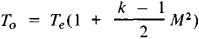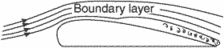# boundary layer

Also found in: Dictionary, Thesaurus, Medical, Acronyms, Wikipedia.
Related to boundary layer: Atmospheric boundary layer

## boundary layer

[′bau̇n·drē ‚lā·ər]
(meteorology)
The lower portion of the atmosphere, extending to a height of approximately 1.2 miles (2 kilometers).

## Boundary Layer

in a viscous fluid the flow region that forms at the surface of a body past which the fluid moves or at the interface of two streams of fluids with different velocities, temperatures, or chemical compositions; its transverse thickness is much smaller than its longitudinal dimensions. The boundary layer is characterized by an abrupt change in the transverse direction of velocity (a hydrodynamic boundary layer), temperature (a thermal boundary layer), or concentrations of individual chemical components (a diffusion boundary layer). The viscosity, thermal conductivity, and diffusivity of the fluid are the principal influences on the formation of the flow in a boundary layer. Within a dynamic boundary layer a smooth change in velocity occurs from the velocity of the external stream to zero at the wall as a result of the adhesion of the viscous fluid to the solid surface. Similarly, the temperature and concentration vary smoothly within a boundary layer.

The state of flow in a dynamic boundary layer depends on the Reynolds number Re and may be laminar or turbulent. In laminar flow, individual particles of the fluid follow trajectories whose shape is close to that of the body the fluid is flowing past or of the interface between the two liquid or gaseous media. In turbulent flow, the random, pulsating motion of individual particles of the fluid is superimposed on the average motion of the fluid particles in the direction of the main stream. The rate of transfer of momentum and the intensity of the processes of heat and mass transfer consequently are sharply increased, and an increase in skin friction and heat and mass transfer results. The value of the critical Reynolds number, at which the transition from laminar flow to turbulent flow occurs in the boundary layer, depends on several factors, including the degree of roughness of the surface past which the fluid is moving, the level of turbulence of the external stream, and the Mach number M. Moreover, the transition from laminar to turbulent flow with increasing Re in the boundary layer does not occur abruptly; there is a transition region where laminar and turbulent flows alternate.

The thickness δ of a hydrodynamic boundary layer is defined as the distance from the surface of the body (or from the interface of fluids) at which the velocity in the boundary layer can be considered essentially equal to the velocity in the external stream. The value of δ depends chiefly on the Reynolds number. For laminar flow, δ ~ lRe-0.5, and for turbulent flow, δ ~ IRe~-0.2, where l is a reference length of the body.

The development of a thermal boundary layer is determined not only by the Reynolds number but also by the Prandtl number, which characterizes the relationship between the thicknesses of the dynamic and the thermal boundary layers. The Schmidt number correspondingly has an additional effect on the development of a diffusion boundary layer.

When the external stream of gas has a high velocity, a conversion of the kinetic energy of molecules in the boundary layer into thermal energy occurs. As a result, the local temperature of the gas rises. In the case of a thermally insulated surface the temperature of the gas in a boundary layer may approach the stagnation temperaturewhere Te is the gas temperature outside the boundary layer and k = Cp/cv is the ratio of the specific heats at constant pressure and volume.

The character of the flow in a boundary layer has a decisive effect on the separation of the flow from the surface of the body past which the fluid is moving. The reason for this is that when a sufficiently great positive longitudinal pressure gradient is present, the kinetic energy of the fluid particles that are slowed down in the boundary layer becomes insufficient to overcome the pressure forces, the flow in the boundary layer loses stability, and separation of flow occurs.

When the Reynolds numbers are very large, the thickness of the boundary layer is very small in comparison with the characteristic dimensions of the body. Throughout nearly the entire flow region, with the exception of the thin boundary layer, the effect of viscous forces is therefore insignificant in comparison with inertial forces, and the fluid in this region can be regarded as perfect. At the same time, because of the small thickness of the boundary layer, the pressure in it in a transverse direction can be considered essentially constant. As a result, a highly effective method of studying the flow of a fluid stream past a body is the division of the entire flow field into two parts—the flow region of the perfect fluid and the thin boundary layer at the surface of the body. The flow in the first region is studied by means of the equations of motion of a perfect fluid; this approach permits determination of the pressure distribution along the surface of the body. The pressure in the boundary layer is also thereby determined. The flow within the boundary layer is then calculated, taking into account viscosity, thermal conductivity, and diffusion. As a result, the surface friction and the coefficients of heat and mass transfer can be determined. This approach, however, is inapplicable in its explicit form in the case of flow separation from the surface of the body. It also is inapplicable for small Re, when the effect of viscosity extends to quite large distances from the surface of the body.

### REFERENCES

Loitsianskii, L. G. Mekhanika zhidkosti i gaza, 4th ed. Moscow, 1973.
Schlichting, H. Teoriia pogranichnogo sloia. Moscow, 1974. (Translated from German.)
Osnovy teploperedachi v aviatsionnoi i raketnoi tekhnike. Moscow, 1960.
Kutateladze, S. S., and A. I. Leont’ev. Teplomassoobmen i trente v turbulentnom pogranichnom sloe. Moscow, 1972.

N. A. ANFIMOV

## boundary layerThe layer of fluid, close to the surface of a body placed in a moving stream, in which the impact pressure is reduced as a result of the viscosity of the fluid. A velocity gradient exists through the boundary layer, ranging from the velocity of the body to the velocity of the free airstream. The nature of the boundary layer determines the maximum lift coefficient, the stalling characteristics of a wing, the value of form drag, and to an extent the high-speed characteristics. In aerodynamics, the boundary-layer thickness is measured from the surface to an arbitrarily chosen point (e.g., where the velocity is 99% of the stream velocity). Thus, in aerodynamics, the boundary layer, by selection of the reference point, can include only the laminar boundary layer or the laminar boundary layer plus all, or a portion of, the turbulent boundary layer.
References in periodicals archive ?
In the process of sulfuric acid corrosion for concrete, OH- diffuses from interior to exterior of concrete under the action of concentration gradient and reacts with [H.sup.+] in the soaking solution to form the reaction boundary layer. Only when the O[H.sup.-] of the concrete surface has been consumed by [H.sup.+] in the soaking solution, the excess of [H.sup.+] continue to spread to the inner concrete in the concentration gradient effect and further react with OH- inner concrete.
Then, one set of permanently installed L-band radiosonde radar and one GPS sounding system (located next to the MS1) were applied in the atmospheric boundary layer soundings for a selected case study.
An experimental investigation of boundary layer ingestion in a diffusing S-Duct with and without passive flow control, 37th Aerospace Sciences Meeting and Exhibit, Aerospace Sciences Meetings, Reno, NV, USA.
After evaluating the order of magnitude analysis on y-direction momentum Equation (3) which is normal to the sheet and boundary layer approximations in Equations (3)-(5) and (10) define as:
In this paper, a high-order accurate finite difference method is used to simulate the hypersonic flow field over the blunt wedge with a smooth wall and rough wall, and the effect of roughness on the flow state in the boundary layer is discussed.
Rheological equation of nanoscale porous media fluid in boundary layer has been revised based on the Navier-Stokes equation considering the viscosity coefficient formula of boundary layer fluid.
In order to measure the boundary layer thickness behind the tangential blowers in the empty tunnel, a rake of total pressure probes was mounted on the wind tunnel traversing gear and positioned such that the first probe was located 2 mm above the tunnel floor.
At present, an analytical evidence for two-dimensional boundary layer flow over a wedge with an embedded permeability is not yet reported and that requires a credible mechanism for its analysis.
Much later, melting heat transfer in a nanofluid boundary layer on a stretching circular cylinder was examined by Gorla et al.
Luther does take a naturalist's look at the mosses, fungi and lichens that occupy the terrestrial boundary layer, and it is a good introduction to these often overlooked plants.
The classical concept of boundary layer corresponds to a thin region next to the wall in a flow where viscous forces are important which may affect the engineering process of producing.

Site: Follow: Share:
Open / Close